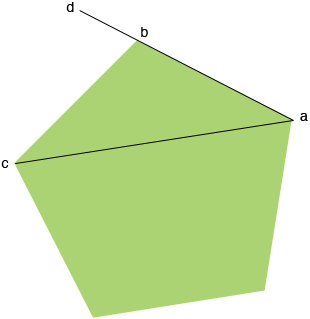SEARCH HOMEMath Central Quandaries & QueriesQuestion from jacob, a student: hello. i am trying to draw a template for a project. but i don't have a protractor. i'm trying to draw a normal pentagon with 2cm sides. what i'm trying to figure out is the distance in centimeters from point a, to c, in a straight line. this is needed so that i can measure and create the shape without a protractor, and keep the angles and sides correct. if you can help me with the measurement, or help me another way, any help would be greatly appreciated. thank you.Hi Jacob,

I can help you get started. Write back if you need more help.

I drew the diagram you described and extended the side ab to a point d.The angle cbd is an exterior angle of the pentagon. For any convex polygon the sum of the exterior angles is $360^o .$ What is the measure of the angle cbd?

Penny

Jacob,

My colleague Chris commented on my response to you. He said

"I saw Penny's answer, but it's a long way from that to what jacob wanted -- he said he doesn't have a protractor, and to get the measure of the diagonal ac from knowing the angle at a vertex requires some facility with geometry. Why not simply tell him to multiply 2cm (which is the side length) by the golden section (1 + sqrt 5)/2 which is approximately 1,618."

PennyMath Central is supported by the University of Regina and The Pacific Institute for the Mathematical Sciences.